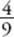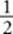﻿ ﻿Exercises - Solid Geometry - SOLID AND COORDINATE GEOMETRY - SAT SUBJECT TEST MATH LEVEL 1

## SOLID AND COORDINATE GEOMETRY## CHAPTER 12 Solid Geometry### Exercises1. What is the volume of a cube whose surface area is 54?

(A) 27

(B) 36

(C) 54

(D) 64

(E) 216

2. What is the surface area of a cube whose volume is 216?

(A) 6

(B) 36

(C) 72

(D) 144

(E) 216

3. What is the volume of a sphere whose surface area is 36π?

(A)(B) 9π

(C) 36π

(D) 72π

(E) 108π

4. What is the surface area of a sphere whose volume is 288π?

(A) 6π

(B) 36π

(C) 72π

(D) 144π

(E) 288π

5. What is the lateral surface area of a right circular cone whose radius is 3 and whose height is 4?

(A) 12π

(B) 15π

(C) 24π

(D) 30π

(E) 36π

6. The height, h, of a right circular cylinder is equal to the edge of a cube. If the cylinder and cube have the same volume, what is the radius of the cylinder?

(A)(B)(C)(D)(E) πh2

7. What is the volume of a pyramid whose base is a square of area 36 and whose four faces are equilateral triangles?

(A) 24(B) 36

(C) 36(D) 108

(E) 1088. An isosceles right triangle whose legs are 6 is rotated about one of its legs to generate a right circular cone. What is the volume of that cone?

(A) 48(B) 72π

(C) 72(D) 144π

(E) 216π

9. If the height of a right circular cylinder is 4 times its circumference, what is the volume of the cylinder in terms of its circumference, C ?

(A)(B)(C)(D)(E) 4πC 3

10. Three identical balls fit snugly into a cylindrical can: the radius of the spheres equals the radius of the can, and the balls just touch the bottom and top of the can. What fraction of the volume of the can is taken up by the balls?

(A)(B)(C)(D)(E)1. (A) 5. (B) 9. (A) 2. (E) 6. (A) 10. (D) 3. (C) 7. (C) 4. (D) 8. (B)

Solutions

Each of the problems in this set of exercises is typical of a question you could see on a Math 1 test. When you take the model tests in this book and, in particular, when you take the actual Math 1 test, if you get stuck on questions such as these, you do not have to leave them out—you can almost always answer them by using one or more of the strategies discussed in the “Tactics” chapter. The solutions given here do not depend on those strategies; they are the correct mathematical ones.

See Important Tactics for an explanation of the symbol ⇒, which is used in several answer explanations.

1. (A) Since the surface area is 54, each of the 6 faces is a square whose area is 546 = 9. So the edges are all 3, and by KEY FACT K1, the volume is 33 = 27.2. (E) Since the volume of the cube is 216, we have e3 = 216e = 6. By KEY FACT K2, the surface area is 6e 2 = 6 x 62 = 6 x 36 = 216.3. (C) Since the surface area of the sphere is 36π, by KEY FACT K8:4. (D) Since the volume of the sphere is 288π, by KEY FACT K8, we have:5. (B) First sketch the cone.By the Pythagorean theorem, the slant height is 5. The circumference of the circular base is 2π(3) = 6π. So by KEY FACT K6, the lateral surface area is:d =(6π)5 = 15π.

6. (A) Since the volumes are equal, πr 2h = e 3 = h 3. So:7. (C) Sketch the pyramid.Since the area of the square is 36, each side is 6; and since each triangular face is equilateral, each edge of the pyramid is 6. To find the height of the pyramid, use the Pythagorean theorem onPED. Sinceis half of diagonal,. So:Finally, by KEY FACT K7, the volume of the pyramid is:8. (B) Sketch the triangle and cone.Since the radius and height of the cone are 6, by KEY FACT K6:9. (A) Since V = πr 2h, we need to express r and h in terms of C. It is given that h = 4C, and since C = 2πr, r =. So:10. (D) The volume of each ball isπr3, and the total volume of the 3 balls is. The height of the can is equal to 3 times the diameter, and hence 6 times the radius, of a ball. So the volume of the can is πr 2(6r) = 6πr 3. Therefore, the balls take upof the can.﻿